SCERT AP 7th Class Maths Solutions Pdf Chapter 11 Area of Plane Figures Ex 11.2 Textbook Exercise Questions and Answers.

## AP State Syllabus 7th Class Maths Solutions 11th Lesson Area of Plane Figures Ex 11.2

Question 1.
The length and breadth of the tablet are 16 cm, 8 cm respectively. It has a inner side black border of 1 cm width around the screen. Find the area of the black border.
Given length of the tablet l = 16 cm
Area of the tablet ABCD
= l × b
= 16 × 8
= 128 sq.cm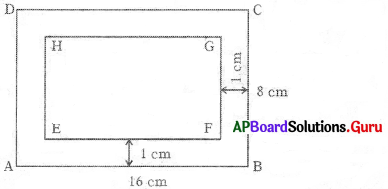Width of border = 1 cm
Border running around the screen.
So, length of screen
l = 16 – 2 × width
= 16 – 2 × 1
= 16 – 2 = 14 cm
b = 8 – 2 × 1
= 8 – 2 = 6 cm
Area of the screen
= l × b
= 14 × 6
= 84 sq.cm
Area of the border = Area of the tablet – Area of screen
= 128 – 84
= 44 sq.cm
∴ Area of black border = 44 sq.cm.Question 2.
Revanth has a rectangular lawn of length 45 m and breadth 20 m in his garden. He wants to do flooring 5 m along out-side the lawn for path. Find out the area of path. Find the cost of flooring at the rate ₹ 100 per sq.m.
Given inner length of lawn l = 45 m
Inner area of lawn = l × b
= 45 × 20
= 900 sq.m
Width of the path = 5 m
Path is outside the lawn.
So, outer length of lawn
L = l + 2 × w
= 45 + 2 × 5
= 45 + 10 = 55 m
Breadth B = b + 2w
= 20 + 2 × 5
= 20 + 10 = 30 m
Outer area of lawn = l × b
= 55 × 30
= 1650 sq.m
Area of the path
= outer area – inner area
= 1650 – 900
= 750 sq.m.
Cost of flooring per sq.m = ₹ 100
∴ Cost of flooring per 750 sq.m
= 750 × 100 = ₹ 75,000/-Question 3.
The surface of water pool is in the shape of a square whose side is 450 cm. Its exterior 20 cm width and the edge part is cemented along the side of the square. Find the area of that cemented part. Find cost for cementing if the rate is ₹ 15 per sq.cm.
Given inner side of the pool (s) = 450 cm
Inner area of waterptool ABCD = s × s
= 450 × 450
= 202500 sq.cm
Width of the edge = 20 cm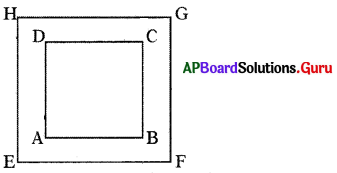Here path is running outside the pool.
So, outer side of the pool
(s) = s + 2 × w
= 450 + 2 × 20
= 450 + 40 = 490 cm
Outer area of waterpool EFGH
= s × s
= 490 × 490
= 2,40,100 sq.cm
Area of the edge
= Outer area – Inner area
= 240100 – 202500
Area of the path = 37,600 sq.cm
Cost of cementing per sq.cm = ₹ 15
∴ Cost of cementing per 37600 sq.cm
= 37,600 × 15
= ₹ 5,64,000Question 4.
Two pathways parallel to the length and breadth have been constructed in the centre of the rectangular park whose length is 120 m and breadth is 90 m. If the width of each pathway is 15 m, find out expenditure of flooring on the pathway at the rate ₹ 80 per sq.m,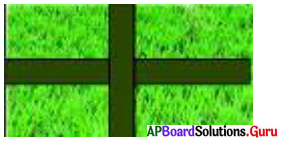In the figure ABCD is a rectangular park.
Length of ABCD = 120 m
Breadth of ABCD = 90 m
Width of path = 15 m
Area of EFGH = length × width
= 120 × 15
= 1800 sq.m
Area of MNOP = breadth × width
= 90 × 15
= 1350 sq.m
Area of common path = width × width
= 15 × 15
= 225 sq.m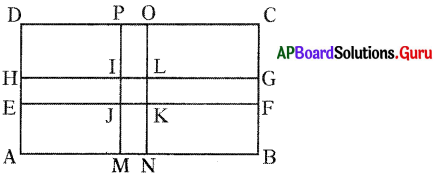Area of IJKL = 225 sq.m is included in both EFGH and MNOP paths. So, we subtract once.
Area of the path = Area of EFGH + Area of MNOP – Area of IJKL .
= 1800 + 1350 – 225
= 2925 sq.m
Cost of flooring per sq.m = ₹ 80
Cost of flooring per 2925 sq.m. = 2925 × 80
∴ Expenditure for path flooring = ₹ 2,34,000.Question 5.
A photo frame of length 28 cm. and breadth 11 cm. has done decoration of 3 cm. along inside shown in the figure. Find the total area of decoration. If the cost of decoration is ₹ 2 per Sq.cm., find total cost of decoration.
Given the length of frame ABCD = 28 cm
Area of frame ABCD = l × b
= 28 × 11
= 308 sq.cm
Width of the decoration part = 3 cm
Decoration is tying inside the frame.
So, length of EFGH = l – 2 × width
= 28 – 2 × 3
= 28 – 6 = 22 cm
Breadth = b – 2 × width
= 11 – 2 × 3
= 11 – 6 = 5 cm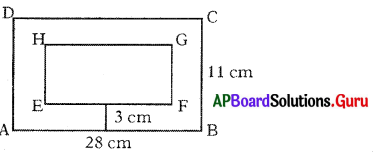Area of frame EFGH = l × b
= 22 × 5
= 110 sq.cm
Area of the decoration part
= Area of ABCD – Area of EFGH
= 308 – 110
= 198 sq.cm
Cost of decoration per sq.cm = ₹ 2
Cost of decoration per 198 sq.cm = 198 × 2
∴ Total cost of decoration = ₹ 396.